# 2. 模糊集：不精确性的传播

## 0x1：扩展 -- 模糊集理论下的不精确性传播方法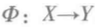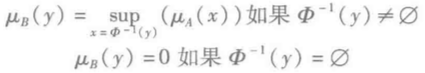## 0x2：扩展原理在笛卡尔乘积中的一个应用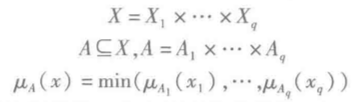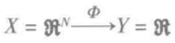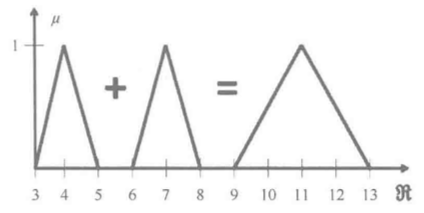# 3. 概率和可能性：针对不确定性的同样方法

## 0x2：似然性扩展原理在笛卡尔乘积中的一个应用

### 1、概率方面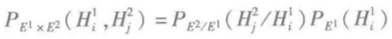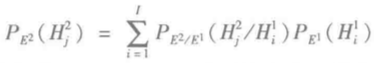### 2、可能性方面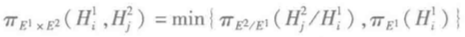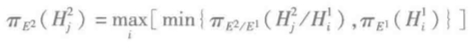• 尺寸
• 形状
• 颜色
• ...

# 5. 扩展运算的应用实例：随时间推移的知识更新

## 0x1：问题背景：地面图像识别

• 沙土
• 水域
• 田地
• 森林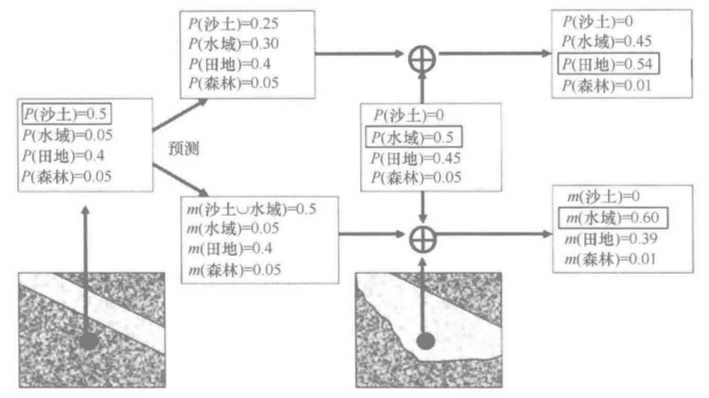P(沙土)=0.5；P(水域)=0.05；P(田地)=0.4；P(森林)=0.05

P(沙土)=0；P(水域)=0.5；P(田地)=0.45；P(森林)=0.05

## 0x2：概率论视角下如何解决这类信源融合问题

• 预测：第一幅图的诠释投射到第二幅图的日期，考虑到最初是沙土的现在是沙土或者水域各自的概率。因此，0.5的沙土概率在两种可能性之间等分，成为水域0.25和沙土0.25，得出第一幅图的诠释在第二幅图的可能预测：P(沙土)=0.25；P(水域)=0.3；P(田地)=0.4；P(森林)=0.05。
• 通过融合从第一幅图得出的预测与第二幅图的实际观察信息，对概率估计进行更新，产生最后的结果：P(沙土)=0；P(水域)=0.45；P(田地)=0.54；P(森林)=0.01

## 0x3：D-S理论视角下如何解决这类信源融合问题

•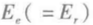：是四个可能类的集合，考虑拍摄第一幅图的时刻
• Es 是同一集合，考虑拍摄第二幅图的时刻
•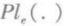：是贝叶斯简单集成了产生于第一幅图的诠释的概率
•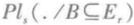：具有 0 或 1 值，解释以下可能的转换的原因：
•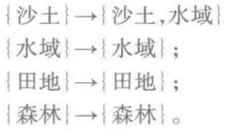• 预测：第一幅图的诠释投射到第二幅图的日期，考虑到最初是沙土的现在是沙土或者水域的概率。扩展运算应用于上述数据只不过导致沙土上的质量分布0.5转移到主要元素（沙土∪水域），以得出第一幅图的诠释在第二幅图上的预测：m(沙土∪水域)=0.5；m(水域)=0.05；m(田地)=0.4；m(森林)=0.05。
• 通过合取组合，从第一幅图像得出的预测与第二幅图的诠释实现更新，产生最后结果：m(沙土)=0；m(水域)=0.6；m(田地)=0.39；m(森林)=0.01。

posted @ 2020-06-10 16:26  郑瀚Andrew.Hann  阅读(70)  评论(0编辑  收藏